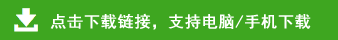# 2020年小学二年级数学下册最新综合练习及答案

一、我会算。(12分)

36÷9=　　　40÷5=

24÷8=　　　5×9=16÷8=

54÷6=　　　48÷8=

35÷5=9÷9=　　　32+18=

6×7-20=　　　4×9+17=

1.18里面有(　　)个3,54除以9的商是(　　),6与8的积是(　　)。

2.8+22=30,54-30=24,把这两道算式改写成一道算式是(　　　　　　　　　)。

3.写出3个成轴对称图形的汉字(　　)、(　　)、(　　)。

4.计算36÷4和36÷9都用(　　　　　　)这句口诀求商。

5.用24根小棒,可以摆(　　)个△或(　　)个□。

6.小明有2元4角钱,如果全部用来买一种商品,那么可以买: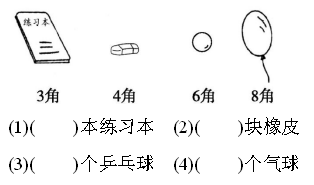7.在○里填上“+”“-”“×”或“÷”。

66=36   　42○6=7

54○6=3○3     12○36=48

70○30=40      6○2=9○3

8.先填空,再列出综合算式。算式:

1.8÷8和8-8的结果相等。(　　)

2.把27分成6份,每份是3。(　　)

3.钟面上时针和分针的转动是旋转现象。(　　)

4.7个7相加是多少,算式是7+7=14。(　　)

5.英语字母“S”“H”“O”都是轴对称图形。(　　)

1.帮小猴分桃。(6分)共有(　　)个桃,平均分给(　　)只小猴,每只小猴分得(　　)个。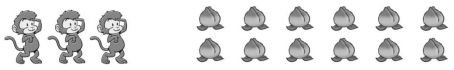□○□=□(个)

2.帮小明分菠萝。(3分)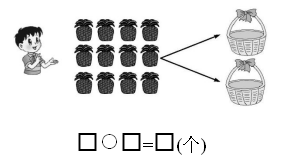3.(3分)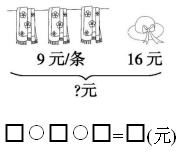1.计算24÷(8-5)时,要先算(　　)。

A.24÷8　　　　　B.8-5

C.24÷8或8-5都可以